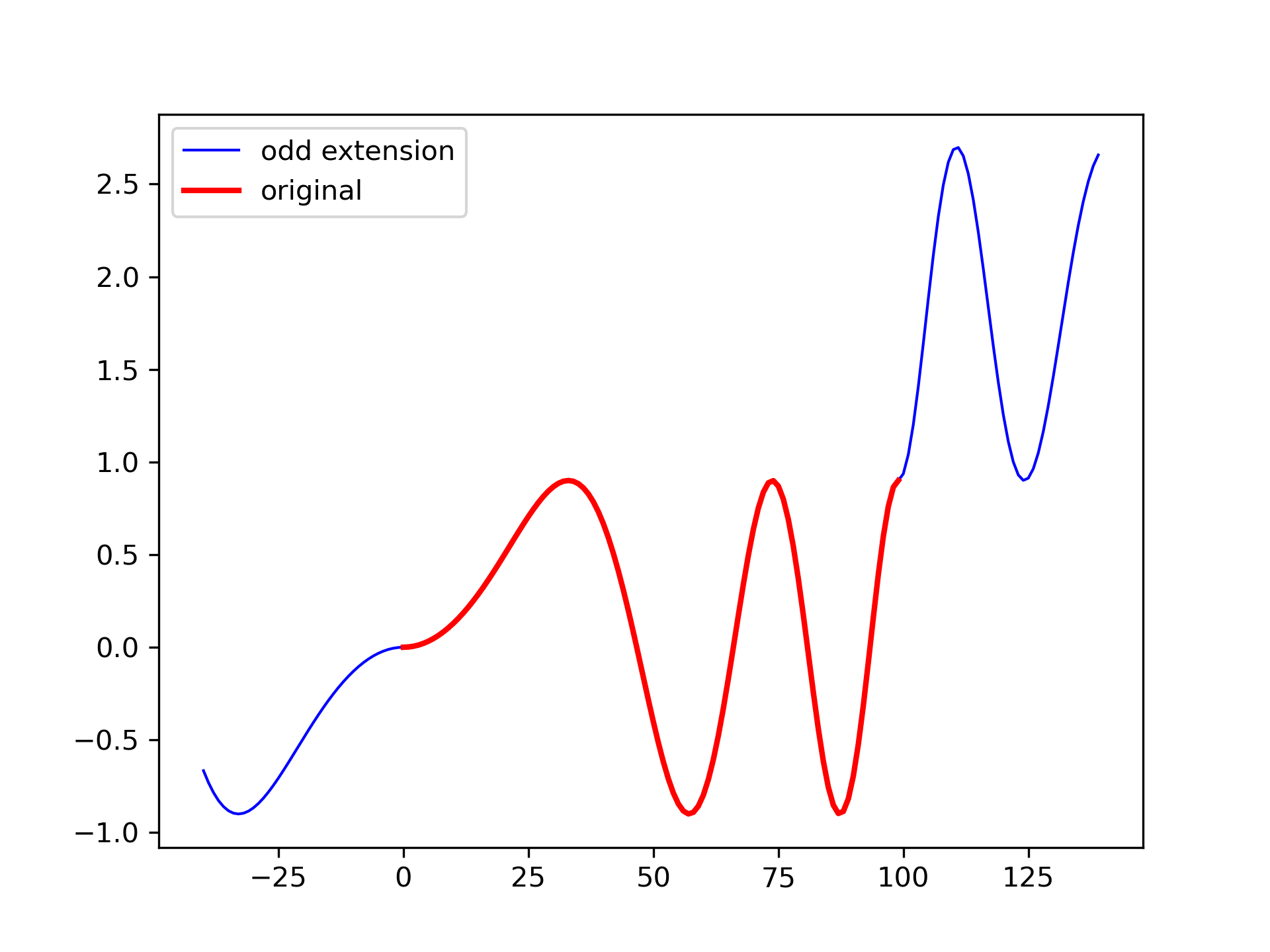scipy 1.8.0 Pypi GitHub Homepage
Other Docs
ParametersBackRef
odd_ext(x, n, axis=-1)

Generate a new ndarray by making an odd extension of x along an axis.

### Parameters

x : ndarray

The array to be extended.

n : int

The number of elements by which to extend x at each end of the axis.

axis : int, optional

The axis along which to extend x. Default is -1.

Odd extension at the boundaries of an array

### Examples

>>> from scipy.signal._arraytools import odd_ext... a = np.array([[1, 2, 3, 4, 5], [0, 1, 4, 9, 16]])... odd_ext(a, 2)
array([[-1,  0,  1,  2,  3,  4,  5,  6,  7],
[-4, -1,  0,  1,  4,  9, 16, 23, 28]])

Odd extension is a "180 degree rotation" at the endpoints of the original array:

>>> t = np.linspace(0, 1.5, 100)... a = 0.9 * np.sin(2 * np.pi * t**2)... b = odd_ext(a, 40)... import matplotlib.pyplot as plt... plt.plot(arange(-40, 140), b, 'b', lw=1, label='odd extension')... plt.plot(arange(100), a, 'r', lw=2, label='original')... plt.legend(loc='best')... plt.show()See :

### Back References

The following pages refer to to this document either explicitly or contain code examples using this.

scipy.signal._arraytools.odd_ext

### Local connectivity graph

Hover to see nodes names; edges to Self not shown, Caped at 50 nodes.

Using a canvas is more power efficient and can get hundred of nodes ; but does not allow hyperlinks; , arrows or text (beyond on hover)

SVG is more flexible but power hungry; and does not scale well to 50 + nodes.

All aboves nodes referred to, (or are referred from) current nodes; Edges from Self to other have been omitted (or all nodes would be connected to the central node "self" which is not useful). Nodes are colored by the library they belong to, and scaled with the number of references pointing them

GitHub : /scipy/signal/_arraytools.py#55
type: <class 'function'>
Commit: